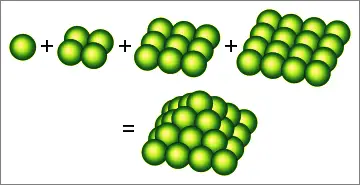# Sum of Consecutive Square Integers & Square Pyramidal Numbers

Consecutive Square Sum Calculator
Find the sum of consecutive squares from
2  to  2  (inclusive).

The sum of the first n squares, 1 + 4 + 9 + ... + n², is called the nth square pyramidal number. They are so-called because they can be represented by square pyramid formations.Rather than add the terms individually, you can calculate the sum of any sequence of consecutive squares by using the simple summation formula given below, or the calculator on the left.

### Square Pyramidal Number Formula

Let S(n) be the nth square pyramidal number. S(n) is defined by the expression

S(n) = 1 + 4 + 9 + ... + (n-1)² + n².       n terms

To find an explicit formula for this sum, consider the sum of n consecutive cubes from 2³ to (n+1)³, that is, the sum Σj=1...n(1+j)³.

Σj=1...n(1+j)³ = Σj=1...n(1 + 3j + 3j² + j³)

Σ(1+j)³ - Σj³ = Σ1 + 3Σj + 3Σj²

(1+j)³ - 1 = Σ1 + 3Σj + 3Σj²

(n+1)³ - 1 = n + 3n(n+1)/2 + 3Σj²

(n+1)³ - 1 - n - 3n(n+1)/2 = 3Σj²

n³ + 1.5n² + 0.5n = 3Σj²

n(n+1)(2n+1)/6 = Σj²

Thus, S(n) = n(n+1)(2n+1)/6.

### Sum of Consecutive Squared Integers Formula

The sum of consecutive numbers from a² to b² inclusive is the difference between the bth square pyramidal number and the ath square pyramidal number plus a². (You need to add a² because the sum is inclusive of the endpoints a² and b².)

Example: Find the sum of squares from 100² to 200² inclusive.

S(200) - S(100) + 100²
= 200(201)(401)/6 + 100(101)(201) + 100²
= 2686700 - 338350 + 10000
= 2358350

### Fun Facts About Square Pyramid Numbers

1. The only two square pyramidal numbers that are also squares are 1 and 4900, which is the sum of the first 24 squares.

2. The only square pyramidal numbers that are also triangular numbers are 1, 55, 91, and 208335.

T(1) = 1 = S(1)
T(10) = 55 = S(5)
T(13) = 91 = S(6)
T(645) = 208335 = S(85)

3. The height of a stack of spheres in a square pyramid formation is

d + d(n-1)√1/2,

where d is the diameter of each sphere and n is the number of balls along the bottom edge of the base. See Pyramidal Stack Calculator.

4. The sum of two consecutive tetrahedral (triangular pyramidal) numbers, P(n) + P(n-1), is a square pyramidal number, S(n). Proof:

P(n) + P(n-1) = n(n+1)(n+2)/6 + (n-1)n(n+1)/6

= [n³ + 3n² + 2n]/6 + [n³ - n]/6

= [2n³ + 3n² + n]/6

= n(n+1)(2n+1)/6

= S(n)

5. The infinite sum of the reciprocals of the square pyramidal numbers is 18 - 24ln(2). This can be demonstrated by transforming the sum into an equivalent form as follows:

Σn=1...∞1/S(n) = Σn=1...∞6/[n(n+1)(2n+1)]

= 6Σn=1...∞[1/n + 1/(n+1) - 4/(n+1)]

= 6Σn=1...∞[1/n - 2/(2n+1)] - 6Σn=1...∞[2/(2n+1) - 1/(n+1)]

= 6Σn=1...∞[2/(2n) - 2/(2n+1)] - 6Σn=1...∞[2/(2n+1) - 2/(2n+2)]

= 12Σn=2...∞(-1)n/n + 12Σn=3...∞(-1)n/n

Because the alternating series Σn=1...∞(-1)n/n converges to -ln(2), this is equal to

12(-ln(2) + 1) + 12(-ln(2) + 1/2)

= 18 - 24ln(2)